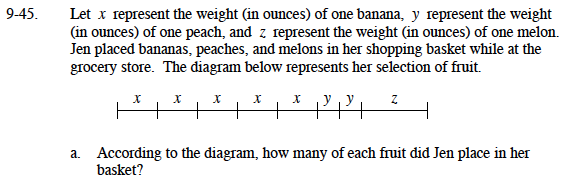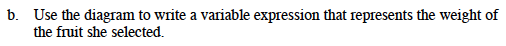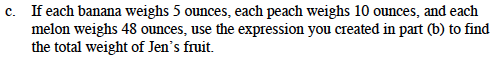### Home > MC1 > Chapter 9 > Lesson 9.1.4 > Problem9-45

9-45.There are 5 x's, 2 y's, and 1 z.If there were 7 x's, 1 y, and 3 z's the expression would look like this: 7x + y + 3zIf your expression was the one in the example in part (b), you would find the total weight of Jen's fruit with this calculation:
7(5 oz) + 10 oz + 3(48 oz).